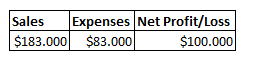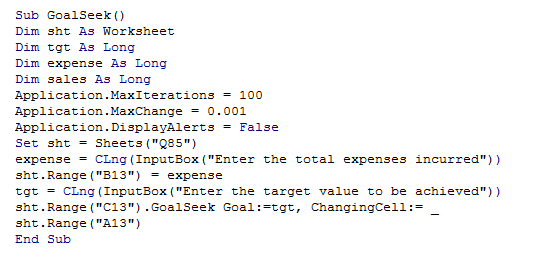## Vba goal seek in vba in Excel

Goal Seek is another tool under "What If" analysis that does a unique function as Scenario Manager.

In Scenario Manager, we predicted the outcomes of various possible Input combinations.

For example, we predicted the Profit of a company based on various assumptions of Sales and Expenses.

The Goal Seek, unlike Scenario Manager, does the exact reverse.

What if one has the desired output, for example, say the same company wants to achieve \$80,000 profit,

but don't know the input values, in this case, sales and expenses?

This is where "Goal Seek" comes for help.

Here, we set the desired output and predict the possible input combinations to achieve this.

This is illustrated in the following exampe.From the above example, let us assume that the company has decided to spend \$75,000 as expense but want to achieve \$80,000 as profit.

Now, let us see how Goal Seek helps with this.

The following code c reates a Goal seek in VBA.

1. Sub GoalSeek()
2. Dim sht As Worksheet
3. Dim tgt As Long
4. Dim expense As Long
5. Dim sales As Long
6. Application.MaxIterations = 100
7. Application.MaxChange = 0.001
9. Set sht = Sheets("Q85")
10. expense = CLng(InputBox("Enter the total expenses incurred"))
11. sht.Range("B13") = expense
12. tgt = CLng(InputBox("Enter the target value to be achieved"))
13. sht.Range("C13").GoalSeek Goal:=tgt, ChangingCell:= _
14. sht.Range("A13")
15. End Sub

When run, the desired sales to be attained is shown in Cell "A13".

The screenshot of the editor is shown below.